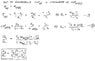# Reversible heat pump - Work input?

• Splint
In summary, the ideal heat engine takes in Q1 heat units at T1 and rejects Q2 at T2. The heat engine then abstracts Q4 from the sink at T4 and discharges Q3 at T3. The efficiency of the heat engine is maximized when the carnot Efficiency is reached.

#### Splint

1. An ideal heat engine (HE) is used to drive a reversible heat pump (HP) as shown in figure
below. The HE takes in Q1 heat units at T1 and rejects Q2 at T2. The HP abstracts Q4 from the sink at T4 and discharges Q3 at T3. Develop an expression for the ratio Q4/Q1 in terms of the four temperatures (T1, T2, T3, & T4)

2.I have sourced some equations for work from elsewhere, but I am unfamiliar with these equations for work as I have not seen them in any of my textbooks or lecture notes. I don't know if the equations are correct, I guess what I'm looking for is confirmation that they are correct and some explanation or reference source so I can understand how these equations were derived.

Thanks

#### Attachments

• HEHP.bmp
61.2 KB · Views: 549
• Work equations.bmp
258.7 KB · Views: 582
If the heat engine is ideal, then the maximum efficiency it can work at the carnot efficiency. Do you know how to express this efficiency in terms of the temperatures?

That would be (Th-Tl)/Th correct?

Splint said:
That would be (Th-Tl)/Th correct?

Right so

ηcarnot= (Th-Tl)/Th = 1 - Tl/Th

What is this for your heat engine? (i.e. in terms of T1 and T3)

ηcarnot is also the same as Work Output/Heat Input

So what is your work output equal to?

Splint said:
That would be (Th-Tl)/Th correct?

Correct.

Use the refrigerator COP of the heat pump (Qc/W) where W is the output of the heat engine: and the efficiency of the heat engine: W/Qh to find the ratio of Q4/Q1 in terms of COP and η. Then all you have to do is express the COP and η in terms of temperatures, T1, T2, T3 and T4.

AM

What is this for your heat engine? (i.e. in terms of T1 and T3)
ηcarnot= (T1-T2)/T1 = 1 - T1/T2 Please note that there appears to be a misprint on the diagram. T3 located by Q2 should be (presumably) T2.

COPr = Q4/(Q3-Q4) = T3/(T4-T3)

Looks like I got it sorted. Thanks for your assistance wit this.

#### Attachments

•Solution.jpg
25.1 KB · Views: 481